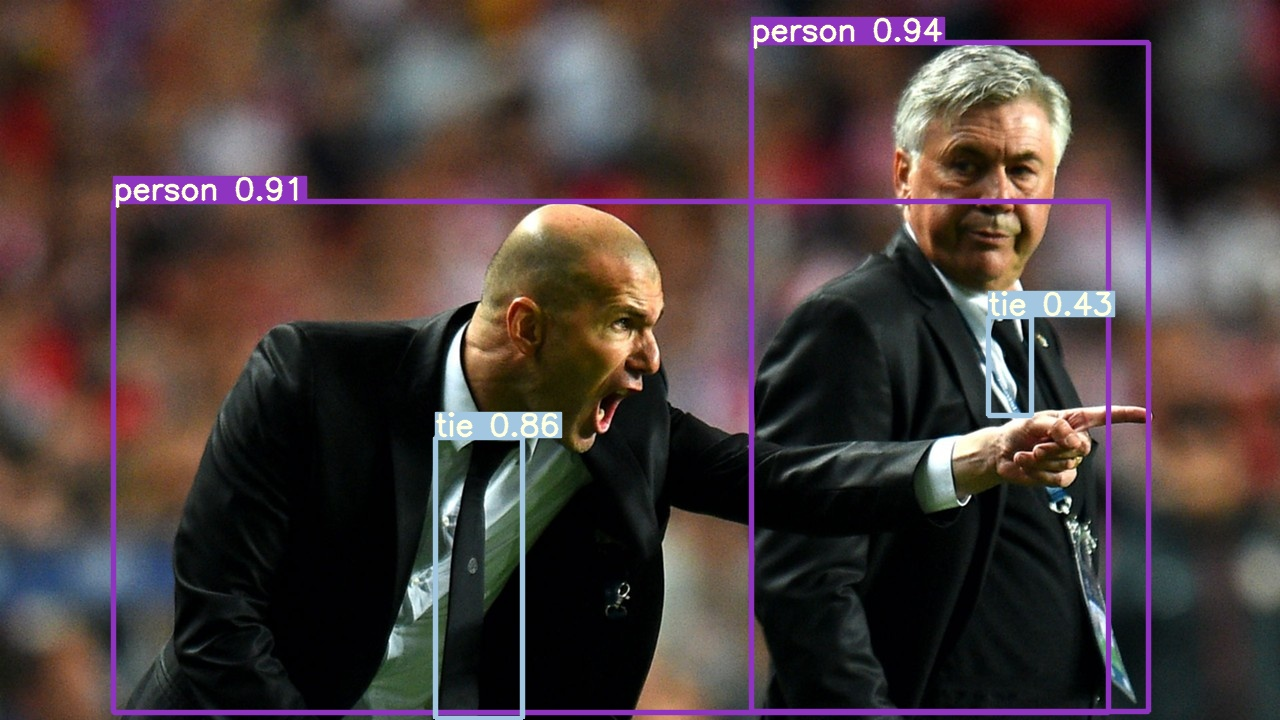Model Ensembling

📚 This guide explains how to use YOLOv5 🚀 model ensembling during testing and inference for improved mAP and Recall. From https://www.sciencedirect.com/topics/computer-science/ensemble-modeling:

Ensemble modeling is a process where multiple diverse models are created to predict an outcome, either by using many different modeling algorithms or using different training data sets. The ensemble model then aggregates the prediction of each base model and results in once final prediction for the unseen data. The motivation for using ensemble models is to reduce the generalization error of the prediction. As long as the base models are diverse and independent, the prediction error of the model decreases when the ensemble approach is used. The approach seeks the wisdom of crowds in making a prediction. Even though the ensemble model has multiple base models within the model, it acts and performs as a single model.

Before You Start

Clone this repo and install requirements.txt dependencies, including Python>=3.8 and PyTorch>=1.7.

git clone https://github.com/ultralytics/yolov5 # clone repo
cd yolov5
pip install -r requirements.txt # install requirements.txt

Test Normally

Before ensembling we want to establish the baseline performance of a single model. This command tests YOLOv5x on COCO val2017 at image size 640 pixels. yolov5x.pt is the largest and most accurate model available. Other options are yolov5s.pt, yolov5m.pt and yolov5l.pt, or you own checkpoint from training a custom dataset ./weights/best.pt. For details on all available models please see our README table.

\$ python test.py --weights yolov5x.pt --data coco.yaml --img 640

Output:

Namespace(augment=False, batch_size=32, conf_thres=0.001, data='./data/coco.yaml', device='', img_size=640, iou_thres=0.65, save_json=True, save_txt=False, single_cls=False, task='val', verbose=False, weights=['yolov5x.pt'])
Using CUDA device0 _CudaDeviceProperties(name='Tesla P100-PCIE-16GB', total_memory=16280MB)

Fusing layers... Model Summary: 284 layers, 8.89222e+07 parameters, 0 gradients
Scanning labels ../coco/labels/val2017.cache (4952 found, 0 missing, 48 empty, 0 duplicate, for 5000 images): 5000it [00:00, 17761.74it/s]
Class      Images     Targets           P           R      mAP@.5  mAP@.5:.95: 100% 157/157 [02:34<00:00,  1.02it/s]
all       5e+03    3.63e+04       0.409       0.754       0.669       0.476
Speed: 23.6/1.6/25.2 ms inference/NMS/total per 640x640 image at batch-size 32

COCO mAP with pycocotools... saving detections_val2017__results.json...
Average Precision  (AP) @[ IoU=0.50:0.95 | area=   all | maxDets=100 ] = 0.492 < ---------- baseline mAP
Average Precision  (AP) @[ IoU=0.50      | area=   all | maxDets=100 ] = 0.676
Average Precision  (AP) @[ IoU=0.75      | area=   all | maxDets=100 ] = 0.534
Average Precision  (AP) @[ IoU=0.50:0.95 | area= small | maxDets=100 ] = 0.318
Average Precision  (AP) @[ IoU=0.50:0.95 | area=medium | maxDets=100 ] = 0.541
Average Precision  (AP) @[ IoU=0.50:0.95 | area= large | maxDets=100 ] = 0.633
Average Recall     (AR) @[ IoU=0.50:0.95 | area=   all | maxDets=  1 ] = 0.376
Average Recall     (AR) @[ IoU=0.50:0.95 | area=   all | maxDets= 10 ] = 0.616
Average Recall     (AR) @[ IoU=0.50:0.95 | area=   all | maxDets=100 ] = 0.670
Average Recall     (AR) @[ IoU=0.50:0.95 | area= small | maxDets=100 ] = 0.493
Average Recall     (AR) @[ IoU=0.50:0.95 | area=medium | maxDets=100 ] = 0.723
Average Recall     (AR) @[ IoU=0.50:0.95 | area= large | maxDets=100 ] = 0.812

Ensemble Test

Multiple pretraind models may be ensembled togethor at test and inference time by simply appending extra models to the --weights argument in any existing test.py or detect.py command. This example tests an ensemble of 2 models togethor: - YOLOv5x - YOLOv5l

\$ python test.py --weights yolov5x.pt yolov5l.pt --data coco.yaml --img 640

Output:

Namespace(augment=False, batch_size=32, conf_thres=0.001, data='./data/coco.yaml', device='', img_size=640, iou_thres=0.65, save_json=True, save_txt=False, single_cls=False, task='val', verbose=False, weights=['yolov5x.pt', 'yolov5l.pt'])
Using CUDA device0 _CudaDeviceProperties(name='Tesla P100-PCIE-16GB', total_memory=16280MB)

Fusing layers... Model Summary: 284 layers, 8.89222e+07 parameters, 0 gradients  # Model 1
Fusing layers... Model Summary: 236 layers, 4.77901e+07 parameters, 0 gradients  # Model 2
Ensemble created with ['yolov5x.pt', 'yolov5l.pt']  # Ensemble Notice

Scanning labels ../coco/labels/val2017.cache (4952 found, 0 missing, 48 empty, 0 duplicate, for 5000 images): 5000it [00:00, 17883.26it/s]
Class      Images     Targets           P           R      mAP@.5  mAP@.5:.95: 100% 157/157 [03:42<00:00,  1.42s/it]
all       5e+03    3.63e+04       0.402       0.764       0.677        0.48
Speed: 37.5/1.4/38.9 ms inference/NMS/total per 640x640 image at batch-size 32

COCO mAP with pycocotools... saving detections_val2017__results.json...
Average Precision  (AP) @[ IoU=0.50:0.95 | area=   all | maxDets=100 ] = 0.496 < ---------- improved mAP
Average Precision  (AP) @[ IoU=0.50      | area=   all | maxDets=100 ] = 0.684
Average Precision  (AP) @[ IoU=0.75      | area=   all | maxDets=100 ] = 0.538
Average Precision  (AP) @[ IoU=0.50:0.95 | area= small | maxDets=100 ] = 0.323
Average Precision  (AP) @[ IoU=0.50:0.95 | area=medium | maxDets=100 ] = 0.548
Average Precision  (AP) @[ IoU=0.50:0.95 | area= large | maxDets=100 ] = 0.633
Average Recall     (AR) @[ IoU=0.50:0.95 | area=   all | maxDets=  1 ] = 0.377
Average Recall     (AR) @[ IoU=0.50:0.95 | area=   all | maxDets= 10 ] = 0.615
Average Recall     (AR) @[ IoU=0.50:0.95 | area=   all | maxDets=100 ] = 0.670
Average Recall     (AR) @[ IoU=0.50:0.95 | area= small | maxDets=100 ] = 0.495
Average Recall     (AR) @[ IoU=0.50:0.95 | area=medium | maxDets=100 ] = 0.723
Average Recall     (AR) @[ IoU=0.50:0.95 | area= large | maxDets=100 ] = 0.815

Ensemble Inference

Append extra models to the --weights argument to run ensemble inference:

\$ python detect.py --weights yolov5x.pt yolov5l.pt --img 640 --source ./inference/images/

Output:

Namespace(agnostic_nms=False, augment=False, classes=None, conf_thres=0.25, device='', img_size=640, iou_thres=0.45, output='inference/output', save_txt=False, source='./inference/images/', update=False, view_img=False, weights=['yolov5x.pt', 'yolov5l.pt'])
Using CUDA device0 _CudaDeviceProperties(name='Tesla P100-PCIE-16GB', total_memory=16280MB)

Fusing layers... Model Summary: 284 layers, 8.89222e+07 parameters, 0 gradients  # Model 1
Fusing layers... Model Summary: 236 layers, 4.77901e+07 parameters, 0 gradients  # Model 2
Ensemble created with ['yolov5x.pt', 'yolov5l.pt']  # Ensemble Notice

image 1/2 inference/images/bus.jpg: 640x512 4 persons, 1 bicycles, 1 buss, Done. (0.073s)
image 2/2 inference/images/zidane.jpg: 384x640 3 persons, 3 ties, Done. (0.063s)
Results saved to inference/output
Done. (0.319s)Environments

YOLOv5 may be run in any of the following up-to-date verified environments (with all dependencies including CUDA/CUDNN, Python and PyTorch preinstalled):

StatusIf this badge is green, all YOLOv5 GitHub Actions Continuous Integration (CI) tests are currently passing. CI tests verify correct operation of YOLOv5 training (train.py), testing (test.py), inference (detect.py) and export (export.py) on MacOS, Windows, and Ubuntu every 24 hours and on every commit.